2021 NFL Offensive Rookie of the Year Odds
+350
3.5 to 1Mac Jones
14.6% implied probability

+550
5.5 to 1Trevor Lawrence
10.1% implied probability

+600
6 to 1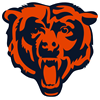Justin Fields
9.4% implied probability

+800
8 to 1Najee Harris
7.3% implied probability

+1000
10 to 1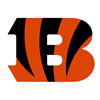Ja'Marr Chase
6.0% implied probability

+1000
10 to 1Trey Lance
6.0% implied probability

+1600
16 to 1DeVonta Smith
3.9% implied probability

+1800
18 to 1Zach Wilson
3.5% implied probability

+2000
20 to 1Elijah Mitchell
3.1% implied probability

No changes have been recorded yet.
+2000
20 to 1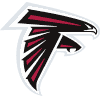Kyle Pitts
3.1% implied probability

+2500
25 to 1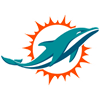Jaylen Waddle
2.5% implied probability

+2500
25 to 1Rondale Moore
2.5% implied probability

+3500
35 to 1Kenneth Gainwell
1.8% implied probability

+3500
35 to 1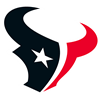Davis Mills
1.8% implied probability

+3500
35 to 1Javonte Williams
1.8% implied probability

+5000
50 to 1Elijah Moore
1.3% implied probability

+5000
50 to 1Trey Sermon
1.3% implied probability

+5000
50 to 1Terrace Marshall
1.3% implied probability

+6500
65 to 1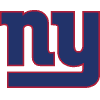Kadarius Toney
1.0% implied probability

+6500
65 to 1Pat Freiermuth
1.0% implied probability

+6500
65 to 1Dyami Brown
1.0% implied probability

+6500
65 to 1Rashod Bateman
1.0% implied probability

+6500
65 to 1Rashawn Slater
1.0% implied probability

+6500
65 to 1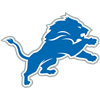Penei Sewell
1.0% implied probability

+8000
80 to 1Amon-Ra St. Brown
0.8% implied probability

+8000
80 to 1Josh Palmer
0.8% implied probability

+8000
80 to 1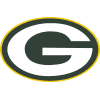Amari Rodgers
0.8% implied probability

+8000
80 to 1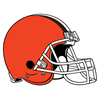Anthony Schwartz
0.8% implied probability

+8000
80 to 1Khalil Herbert
0.8% implied probability

+8000
80 to 1Chris Evans
0.8% implied probability

+10000
100 to 1Tylan Wallace
0.7% implied probability

+10000
100 to 1Sam Ehlinger
0.7% implied probability

+10000
100 to 1Jaelon Darden
0.7% implied probability

+10000
100 to 1Kyle Trask
0.7% implied probability

+10000
100 to 1Brevin Jordan
0.7% implied probability

+10000
100 to 1Tommy Tremble
0.7% implied probability

+10000
100 to 1Cade Johnson
0.7% implied probability

+10000
100 to 1Jamie Newman
0.7% implied probability

+10000
100 to 1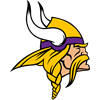Kellen Mond
0.7% implied probability

+10000
100 to 1Alijah Vera-Tucker
0.7% implied probability

+10000
100 to 1Hunter Long
0.7% implied probability

+10000
100 to 1Tutu Atwell
0.7% implied probability